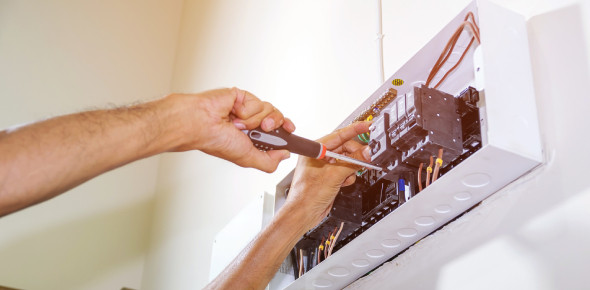# Electricity Test For 8th Grade Student

20 Questions | Attempts: 9803
ShareSettingsHow well do you understand electricity and its uses? Take this electricity test for 8th grade to test yourself on electricity. When the word electricity comes up, several facts and factors come into consideration. You can try this quiz to see how much you know about 8th-grade electricity. This quiz will help you know what your level of understanding is. All the best for a perfect score on this quiz. Do not forget to share the quiz with others.

• 1.
Electric current in a/an ____________________ circuit travels in more than one path.
• A.

Series

• B.

Wire

• C.

Parallel

• D.

Connected

• 2.
A drawing that uses symbols to show how the parts of an electric circuit are connected is a/an _____________________________.
• A.

Nichrome wire

• B.

Electric diagram

• C.

Parallel circuit

• D.

Circuit diagram

• 3.
Which of the following is a good conductor?
• A.

Plastic

• B.

Glass

• C.

Iron

• D.

Wood

• 4.
Which of the following is not a conductor of electricity?
• A.

Iron

• B.

Copper

• C.

Plastic

• D.

Graphite

• 5.
Electric current in a/an _____________________ circuit travels in ONE path.
• A.

Series

• B.

Parallel

• C.

Electric

• D.

Wire

• 6.
A material through which electric current cannot pass easily is a/an ____________________.
• A.

Tester

• B.

Insulator

• C.

Receptor

• D.

Conductor

• 7.
A material through which electric current CAN pass easily in a/an ______________________/
• A.

Tester

• B.

Insulator

• C.

Receptor

• D.

Conductor

• 8.
A fine wire that becomes hot and produces light when an electric current passes through it is called a _________________.
• A.

Filament

• B.

Nichrome wire

• C.

Circuit

• D.

Wire

• 9.
In order for a circuit to work, the path must be __________________.
• A.

Series

• B.

Paralled

• C.

Open

• D.

Closed

• 10.
A/An _____________________ is used to turn the electricity in a current on or off.
• A.

Battery

• B.

Switch

• C.

Conductor

• D.

Insulator

• 11.
The flow of electric charge through something is a/an __________________________.
• A.

Electric current

• B.

Circuit tester

• C.

Battery

• D.

Wire

• 12.
A metal strand used to connect electrical devices in a circuit is a/an ________________________.
• A.

Battery

• B.

Switch

• C.

Filament

• D.

Wire

• 13.
A power source that can produce an electric current as a result of a chemical change in it is a/an ______________________.
• A.

Circuit

• B.

Wire

• C.

Battery

• D.

Filament

• 14.
A circuit must have which of the following?
• A.

• B.

• C.

Energy source, wire, switch

• D.

Battery, wire, switch

• 15.
What is resistance?
• A.

Opposition to current

• B.

Energy from battery

• C.

An increase in current

• D.

An increase in voltage

• 16.
Which device is used to measure current?
• A.

Ammeter

• B.

Voltmeter

• C.

Resistor

• D.

Circuit beaker

• 17.
Which device is used to measure voltage?
• A.

Ammeter

• B.

Voltmeter

• C.

Resistor

• D.

Circuit beaker

• 18.
A low-resistance connection established by accident or intention between two points in an electric circuit is known as _______________.
• A.

Open circuit

• B.

Closed circuit

• C.

Short circuit

• D.

Direct circuit

• 19.
What is the function of a fuse?
• A.

Another name for a switch

• B.

A protective device to slow down the current

• C.

A wire with high resistance to prevent current from passing through

• D.

A protective device for breaking an electric circuit.

• 20.
Which is the correct unit for the current?
• A.

Ohms

• B.

Volts

• C.

Watts

• D.

Amperes

## Related TopicsBack to top
×

Wait!
Here's an interesting quiz for you.